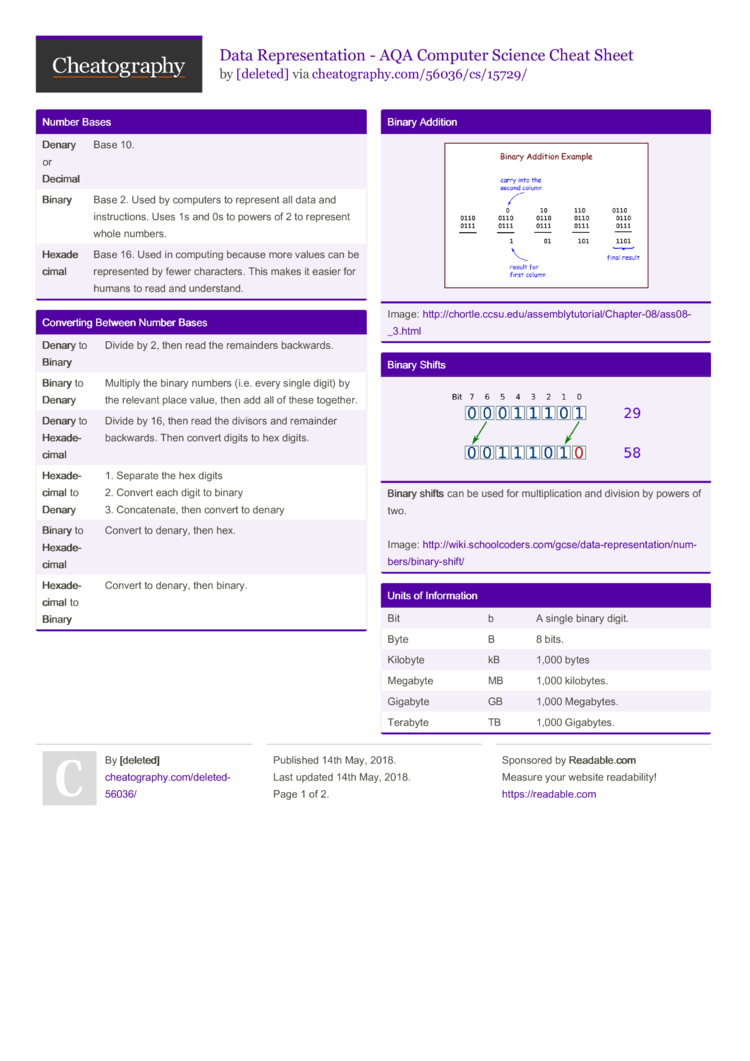# Data Representation - AQA Computer Science Cheat Sheet by [deleted]

### Number Bases

 Denary or Deci­mal Base 10. Binary Base 2. Used by computers to represent all data and instru­ctions. Uses 1s and 0s to powers of 2 to represent whole numbers. Hexa­dec­imal Base 16. Used in computing because more values can be repres­ented by fewer charac­ters. This makes it easier for humans to read and unders­tand.

### Converting Between Number Bases

 Denary to Binary Divide by 2, then read the remainders backwards. Binary to Denary Multiply the binary numbers (i.e. every single digit) by the relevant place value, then add all of these together. Denary to Hexa­dec­imal Divide by 16, then read the divisors and remainder backwards. Then convert digits to hex digits. Hexa­dec­imal to Denary 1. Separate the hex digits 2. Convert each digit to binary 3. Concat­enate, then convert to denary Binary to Hexa­dec­imal Convert to denary, then hex. Hexa­dec­imal to Binary Convert to denary, then binary.

### Binary ShiftsBinary shifts can be used for multip­lic­ation and division by powers of two.

Image: http:/­/wi­ki.s­ch­ool­cod­ers.co­m/g­cse­/da­ta-­rep­res­ent­ati­on/­num­ber­s/b­ina­ry-­shift/

### Units of Inform­ation

 Bit b A single binary digit. Byte B 8 bits. Kilobyte kB 1,000 bytes Megabyte MB 1,000 kilobytes. Gigabyte GB 1,000 Megabytes. Terabyte TB 1,000 Gigabytes.

### ASCII vs Unicode

 What is ASCII?A character set that uses 7 bits, so can represent up to 128 charac­ters; this means that only Latin letters can be used (i.e. charac­ter­s/l­etters from other languages can't be repres­ented). However, it takes up less space that Unicode. What is Unic­ode?Unicode is also a character set, but it can represent many, many values, including non-La­tin­-based languages.

### Repres­enting Images

 Pixel A single point in a graphical issues. Short for 'picture element'. Bitmap A grid of pixels, with each pixel repres­ented by a binary number. Colour depth Number of colours that can (not necess­arily are) be repres­ented in an image, and the corres­ponding number of bits needed to represent each pixel (e.g. 2 bits for 4 colours). The greater the colour depth, the bigger the file size. Reso­lution depth How much detail there is in an image. The more pixels per inch, the higher the resolu­tion. The higher the resolu­tion, the bigger the file size. Meta­data Gives the software the inform­ation needed to display the image properly (size, resolution depth, colour depth).
Bitmap file size = width x height x colour depth (in bits)

### Data Compre­ssion

 Data compre­ssion is used to reduce file size, which means that they take up less storage space. Lossy compre­ssion is where some data is removed - this means that an image would lose some detail. Loss­less compre­ssion preserves all of the inform­ation. Run length encoding (RLE) uses data frequency pairs to reduce the amount of data stored. It does so by stating the character and then the length of the run. Example: 1001 1111 0101 can be shown as 1 1 2 0 5 1 1 0 1 1 1 0 1 1 Huffman coding is more efficient than RLE. It is also lossless. It finds the frequency of each data item to create a Huffman tree, which assigns the most frequent items the shortest code. When you move down a branch to the left, a 0 is assigned. When you move to the right, a 1 is assigned. total bits needed = number of bits needed per character x number of characters

### Repres­enting Sound

 Sample A measure of amplitude at a given point. Used to convert an analogue wave into a digital format. Sampling rate The number of samples taken in a second. Measured in Hertz. Sampling resolu­tion The number of bits per sample. Bit rate The number of bits used per second of the audio. Usually measured in kilobits per second (kbps).
File size (bits) = sampling rate x resolution x lenght of recording (seconds)2 Pages
//media.cheatography.com/storage/thumb/deleted-56036_data-representation-aqa-computer-science.750.jpg

PDF (recommended)